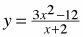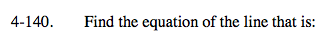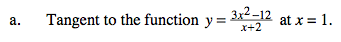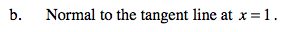### Home > CALC > Chapter 4 > Lesson 4.4.2 > Problem4-140

4-140.
1. Find the equation of the line that is: Homework Help ✎

1. Tangent to the functionat x = 1.

2. Normal to the tangent line at x = 1.Write the equation of the tangent line in point-slope form.

We know that the point of tangency happens at x = 1. Evaluate the function, y, to find the point.
Evaluate the derivative, y', to find the slope.Definition: A 'normal line' is perpendicular to a tangent line at the point of tangency.